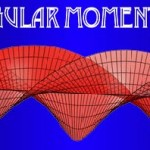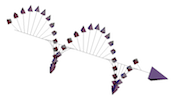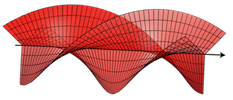## Optical Angular MomentumCircular polarisation

It is well-established in optics that a beam of light can carry both spin and orbital angular momenta in a direction parallel to the beam axis. The spin angular momentum (SAM) is associated with circular polarization in which every polarisation vector rotates. Each photon in a circularly polarised light carries a basic unit of SAM = ±h/2π. The sign of the SAM depends on whether the light is left- or right circularly polarised.Light can also be linearly polarised, which is an equal
superposition of left- and right-circular polarised light. Photons in a linearly polarised light beam thus carry no SAM.Orbital angular momentum; m=3

Orbital angular momentum (OAM) is associated with the presence of helical phase fronts. These beams have an azimuthal phase dependence exp(imφ), where φ is the azimuthal angle and m is the OAM index. The magnitude and sign of m determine the number of intertwined helices and their direction of rotation, respectively (see examples). OAM is quantized – all helically phased beams carry an OAM equivalent to a value of (mh)/2π per photon [Allen 1992].

Together with the Quantum Theory Group at the University of Glasgow, our research in optical angular momentum is divided into two main areas:

• Quantum entanglement in orbital angular momentum
Photons which are entangled in their OAM are of interest for communications as each photon can be encoded to carry much larger amounts of information than if one were using polarization alone. In addition to increasing the information capacity, entangling photons in higher dimensional states can also help reduce the detrimental effect of noise [Tyler].

Quantum Imaging is imaging using quantum correlations, or imaging taking advantage of the quantum properties of light. One type is Ghost Imaging, where coincidence measurements of two beams of entangled photons created in SPDC – one of which interacts with an object and one of which doesn’t – can be used to reconstruct a “ghost” image of the object [Jack].

The edges of the object can be enhanced by using quantum correlations in the OAM of the down-converted photon pairs.

• Optical helicity
A description of optical helicity, its relationship to optical spin, and its potential for a new method of using optical forces to separate chiral molecules. This could have applications in discriminating enantiomers of chiral molecules.